# Selina Concise Chemistry Solution for ICSE Class 10 Chapter 10 Study of Compounds – Nitric Acid

Nitric acid is one of the most important compounds that is used in the manufacture of many industrial as well as commercial products. Thus, it is crucial for science students to study about nitric acid and gain proper knowledge early on. Selina chemistry textbook chapter 10 basically captures the essence of this topic and students mainly learn about the laboratory method of preparation of nitric acid from potassium nitrate or sodium nitrate in this chapter. Furthermore, Concise Chemistry Class 10 ICSE Solutions for Chapter 10 guides students to study the experiments in terms of reactant, equation, setting, product, condition, diagram, precaution, and collection.

## Download PDF Of ICSE Solutions for Class 10 Chemistry Chapter 10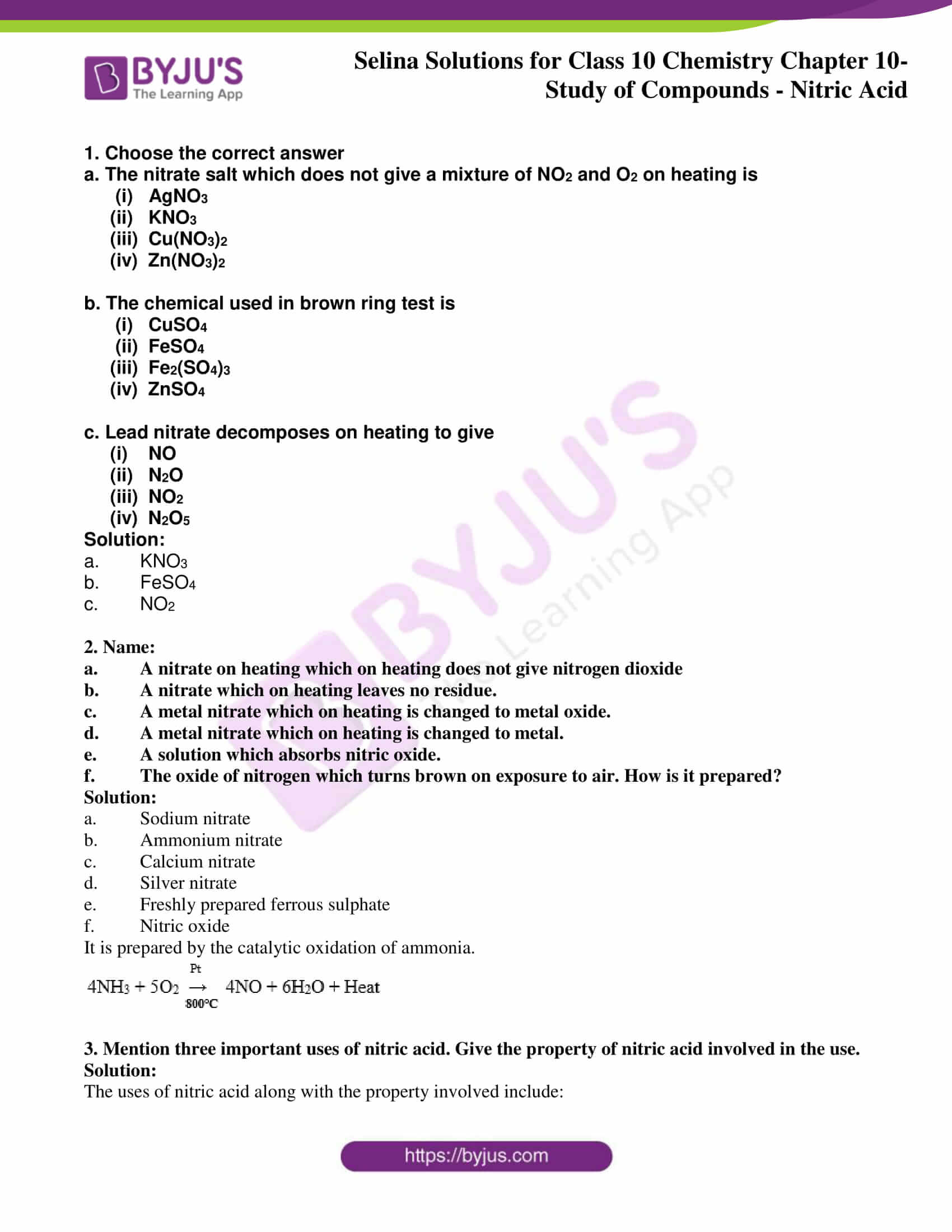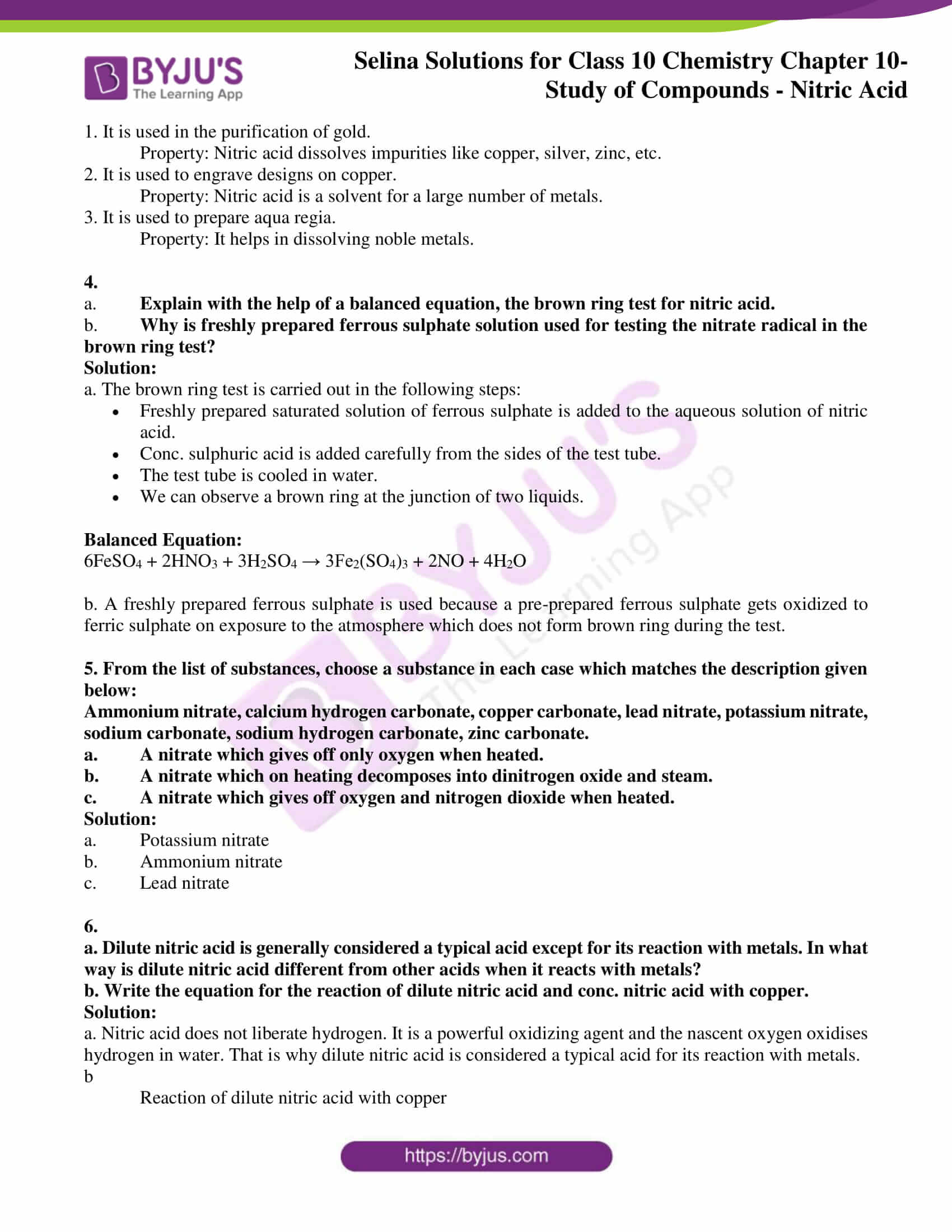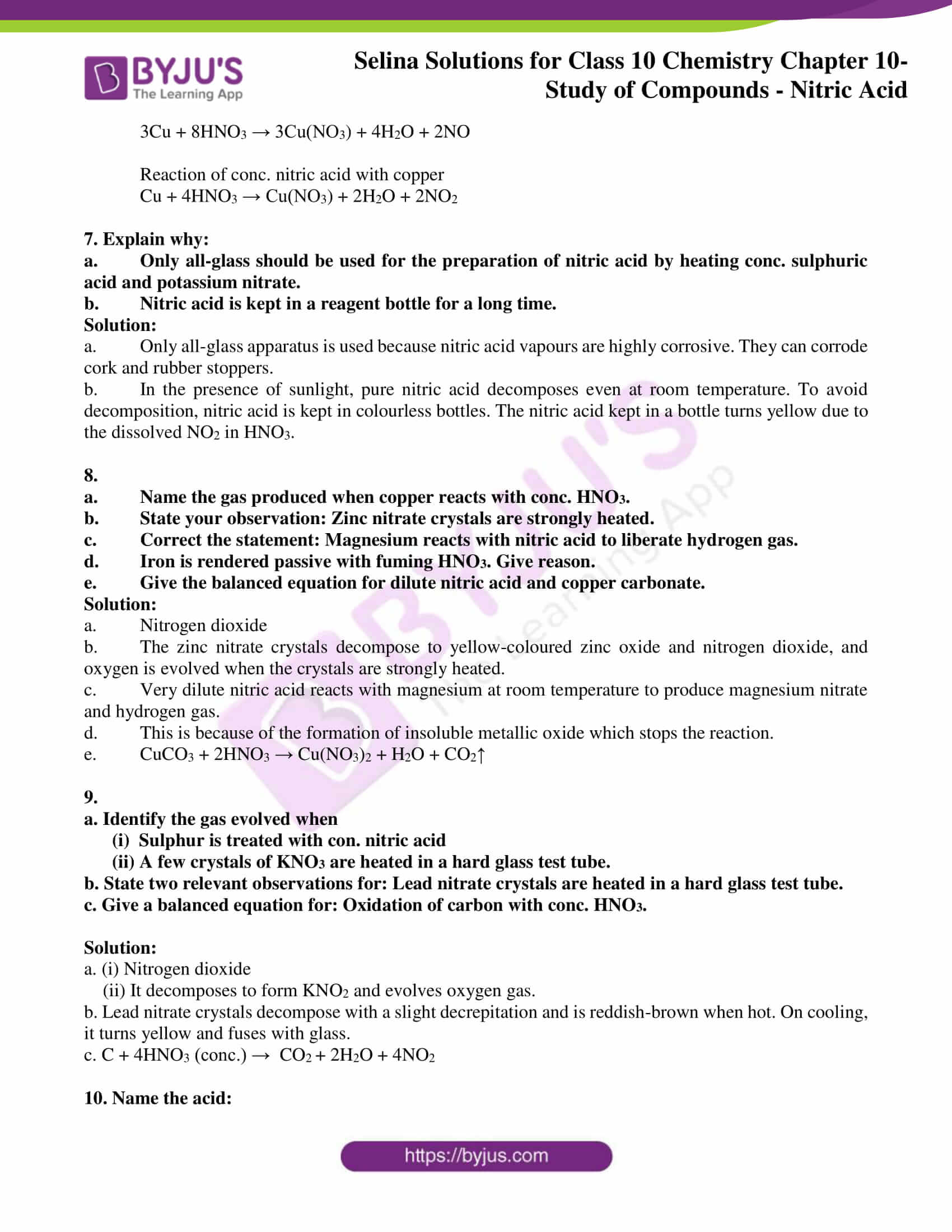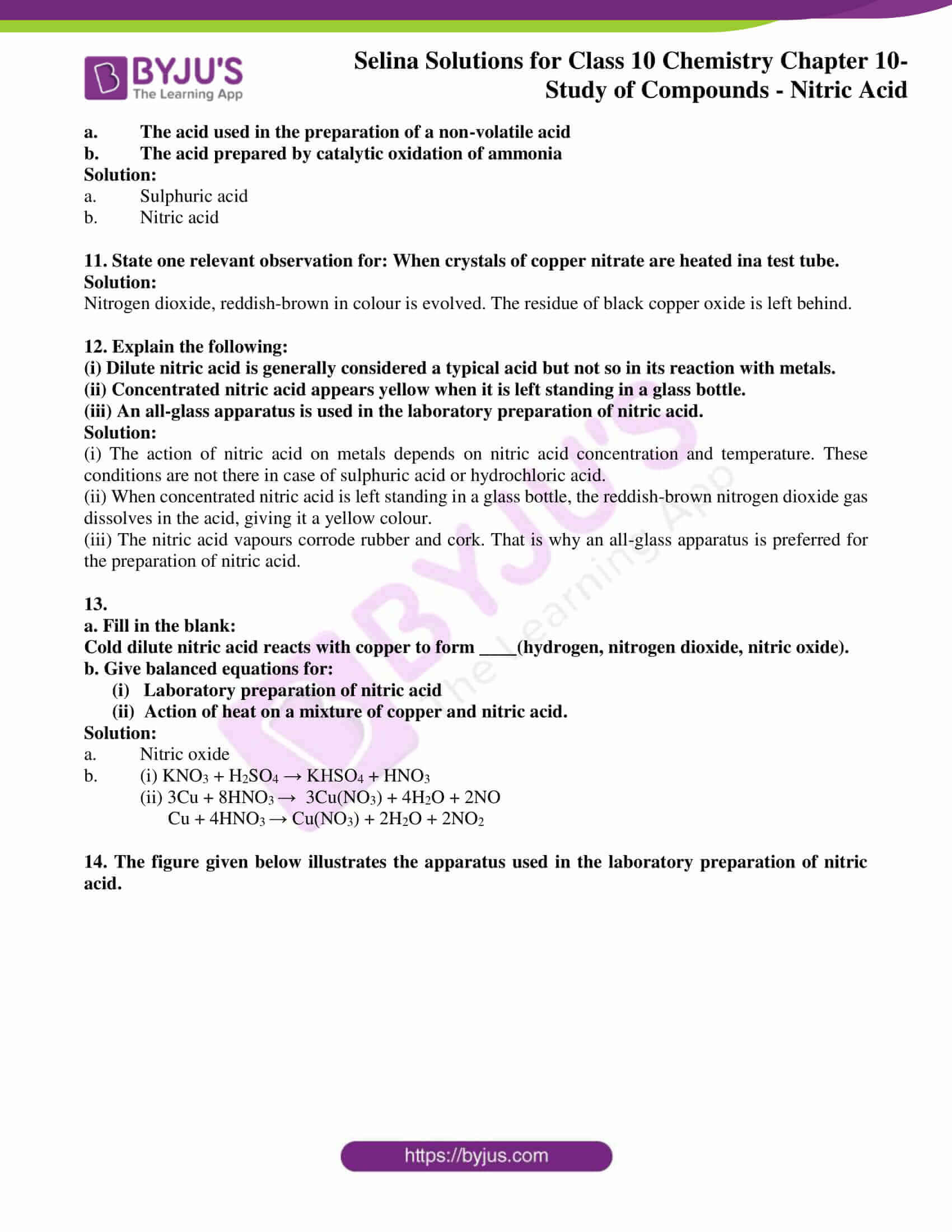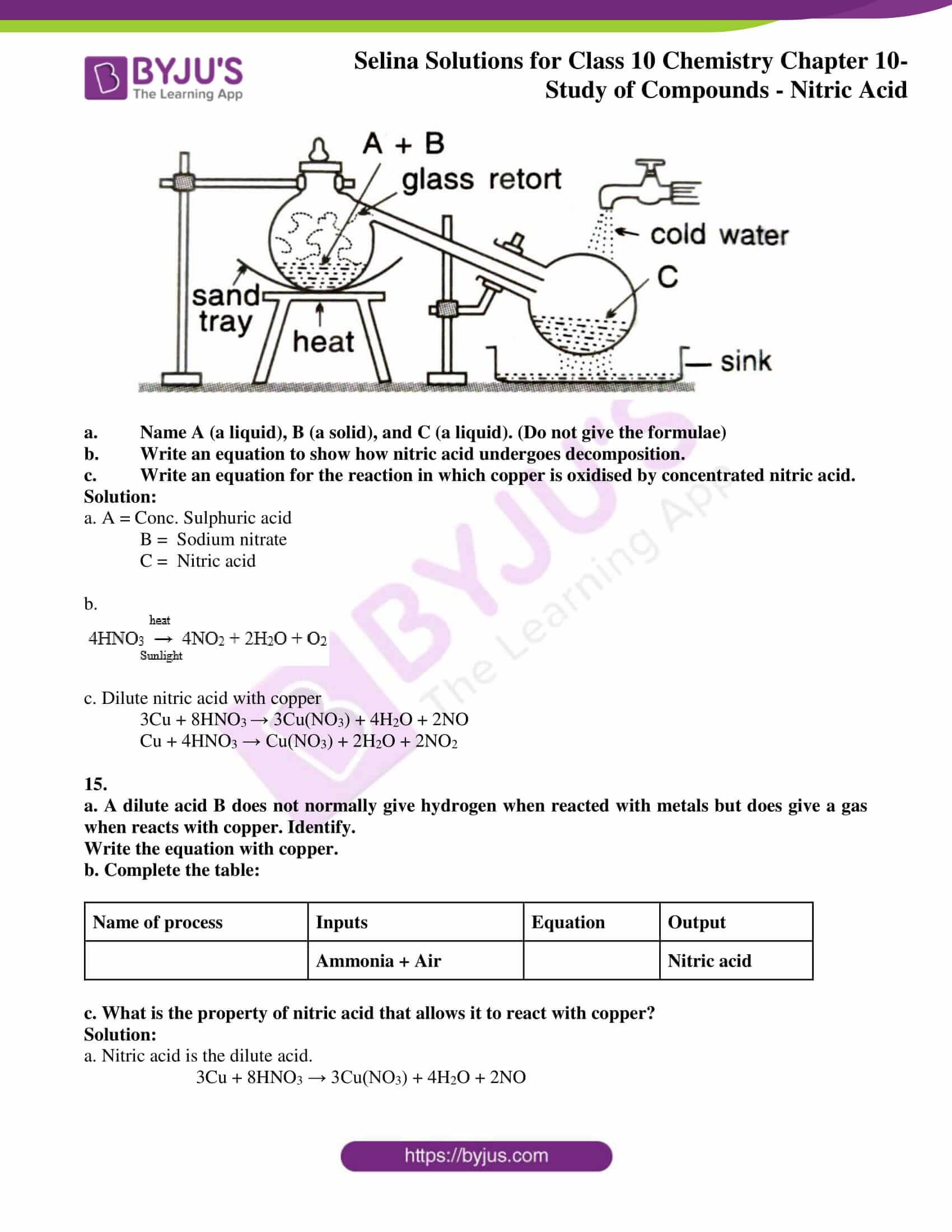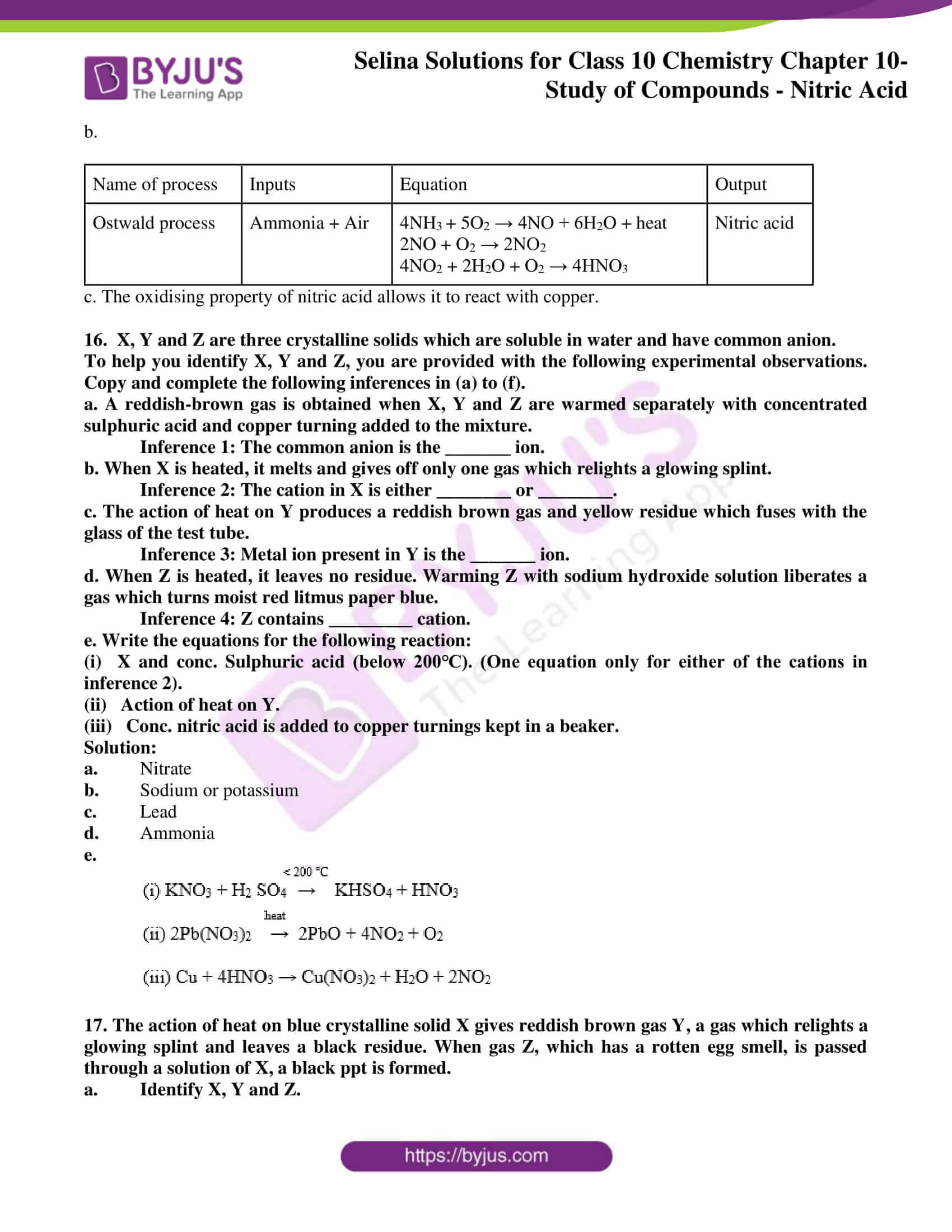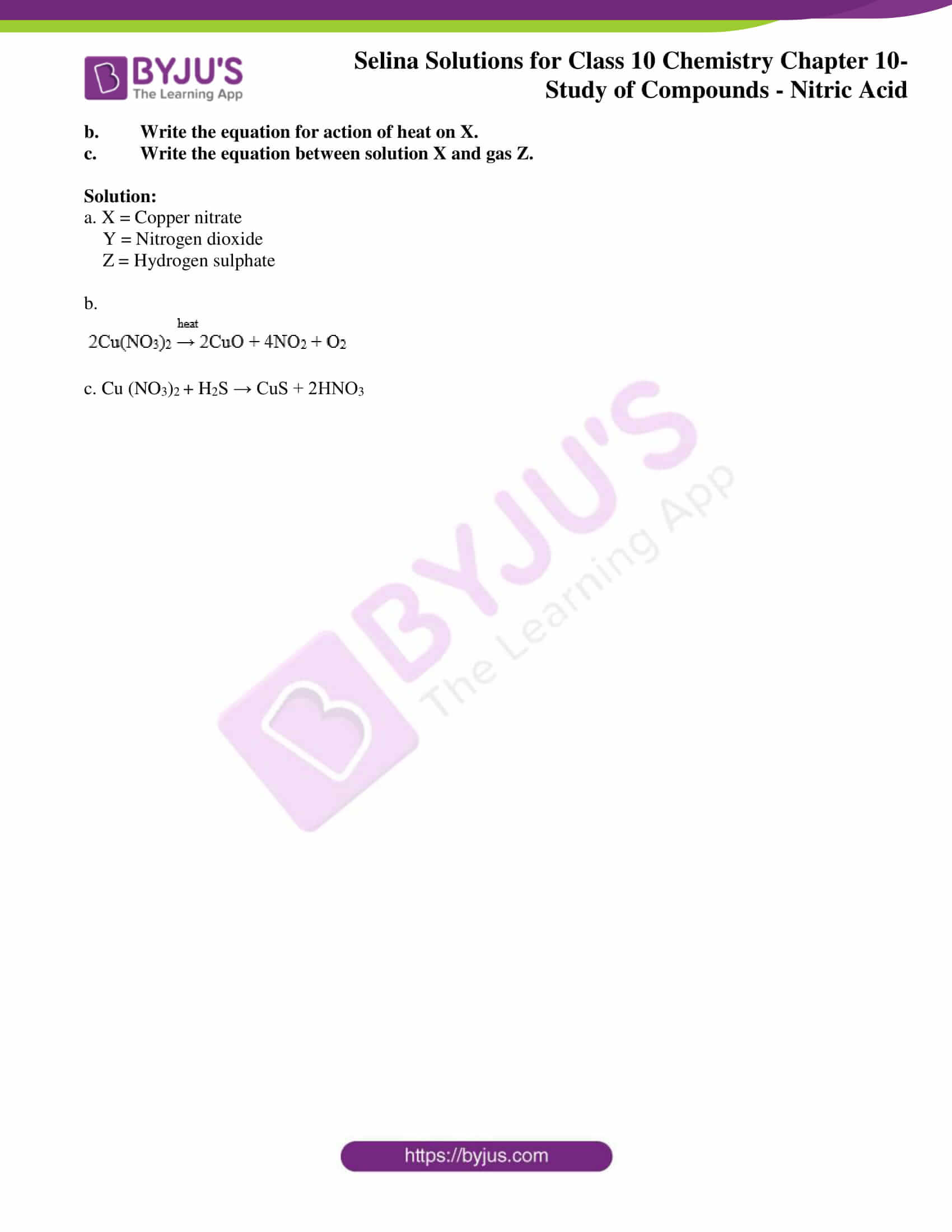### Access ICSE Solutions for Class 10 Chemistry Chapter 10

a. The nitrate salt which does not give a mixture of NO2 and O2 on heating is

(i)   AgNO3

(ii)   KNO3

(iii)  Cu(NO3)2

(iv)  Zn(NO3)2

b. The chemical used in the brown ring test is

(i)   CuSO4

(ii)  FeSO4

(iii)  Fe2(SO4)3

(iv)  ZnSO4

c. Lead nitrate decomposes on heating to give

(i)    NO

(ii)   N2O

(iii)  NO2

(iv)  N2O5

Solution:

1. KNO3
2. FeSO4
3. NO2

2. Name:

1. A nitrate on heating which on heating does not give nitrogen dioxide
2. A nitrate which on heating leaves no residue.
3. A metal nitrate which on heating is changed to metal oxide.
4. A metal nitrate which on heating is changed to metal.
5. A solution which absorbs nitric oxide.
6. The oxide of nitrogen which turns brown on exposure to air. How is it prepared?

Solution:

1. Sodium nitrate
2. Ammonium nitrate
3. Calcium nitrate
4. Silver nitrate
5. Freshly prepared ferrous sulphate
6. Nitric oxide

It is prepared by the catalytic oxidation of ammonia.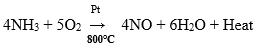3. Mention three important uses of nitric acid. Give the property of nitric acid involved in the use.

Solution:

The uses of nitric acid along with the property involved include:

1. It is used in the purification of gold.

Property: Nitric acid dissolves impurities like copper, silver, zinc, etc.

2. It is used to engrave designs on copper.

Property: Nitric acid is a solvent for a large number of metals.

3. It is used to prepare aqua regia.

Property: It helps in dissolving noble metals.

4.

1. Explain with the help of a balanced equation, the brown ring test for nitric acid.
2. Why is freshly prepared ferrous sulphate solution used for testing the nitrate radical in the brown ring test?

Solution:

a. The brown ring test is carried out in the following steps:

• A freshly prepared saturated solution of ferrous sulphate is added to the aqueous solution of nitric acid.
• Conc. sulphuric acid is added carefully from the sides of the test tube.
• The test tube is cooled in water.
• We can observe a brown ring at the junction of two liquids.

Balanced Equation:

6FeSO4 + 2HNO3 + 3H2SO4 → 3Fe2(SO4)3 + 2NO + 4H2O

b. A freshly prepared ferrous sulphate is used because a pre-prepared ferrous sulphate gets oxidized to ferric sulphate on exposure to the atmosphere which does not form brown ring during the test.

5. From the list of substances, choose a substance in each case which matches the description given below:

Ammonium nitrate, calcium hydrogen carbonate, copper carbonate, lead nitrate, potassium nitrate, sodium carbonate, sodium hydrogen carbonate, zinc carbonate.

1. A nitrate which gives off only oxygen when heated.
2. A nitrate which on heating decomposes into dinitrogen oxide and steam.
3. A nitrate which gives off oxygen and nitrogen dioxide when heated.

Solution:

1. Potassium nitrate
2. Ammonium nitrate

6.

a. Dilute nitric acid is generally considered a typical acid except for its reaction with metals. In what way is dilute nitric acid different from other acids when it reacts with metals?

b. Write the equation for the reaction of dilute nitric acid and conc. nitric acid with copper.

Solution:

a. Nitric acid does not liberate hydrogen. It is a powerful oxidizing agent and the nascent oxygen oxidises hydrogen in water. That is why dilute nitric acid is considered a typical acid for its reaction with metals.

b

The reaction of dilute nitric acid with copper

3Cu + 8HNO3 → 3Cu(NO3) + 4H2O + 2NO

The reaction of conc. nitric acid with copper

Cu + 4HNO3 → Cu(NO3) + 2H2O + 2NO2

7. Explain why:

1. Only all-glass should be used for the preparation of nitric acid by heating conc. sulphuric acid and potassium nitrate.
2. Nitric acid is kept in a reagent bottle for a long time.

Solution:

1. Only all-glass apparatus is used because nitric acid vapours are highly corrosive. They can corrode cork and rubber stoppers.
2. In the presence of sunlight, pure nitric acid decomposes even at room temperature. To avoid decomposition, nitric acid is kept in colourless bottles. The nitric acid kept in a bottle turns yellow due to the dissolved NO2 in HNO3.

8.

1. Name the gas produced when the copper reacts with conc. HNO3.
2. State your observation: Zinc nitrate crystals are strongly heated.
3. Correct the statement: Magnesium reacts with nitric acid to liberate hydrogen gas.
4. Iron is rendered passive with fuming HNO3. Give reason.
5. Give the balanced equation for dilute nitric acid and copper carbonate.

Solution:

1. Nitrogen dioxide
2. The zinc nitrate crystals decompose to yellow-coloured zinc oxide and nitrogen dioxide, and oxygen is evolved when the crystals are strongly heated.
3. Very dilute nitric acid reacts with magnesium at room temperature to produce magnesium nitrate and hydrogen gas.
4. This is because of the formation of insoluble metallic oxide which stops the reaction.
5. CuCO3 + 2HNO3 → Cu(NO3)2 + H2O + CO2

9.

a. Identify the gas evolved when

(i)  Sulphur is treated with con. nitric acid

(ii) A few crystals of KNO3 are heated in a hard glass test tube.

b. State two relevant observations for: Lead nitrate crystals are heated in a hard glass test tube.

c. Give a balanced equation for Oxidation of carbon with conc. HNO3.

Solution:

a. (i) Nitrogen dioxide

(ii) It decomposes to form KNO2 and evolves oxygen gas.

b. Lead nitrate crystals decompose with a slight decrepitation and is reddish-brown when hot. On cooling, it turns yellow and fuses with glass.

c. C + 4HNO3 (conc.) →  CO2 + 2H2O + 4NO2

10. Name the acid:

1. The acid used in the preparation of a non-volatile acid
2. The acid prepared by catalytic oxidation of ammonia

Solution:

1. Sulphuric acid
2. Nitric acid

11. State one relevant observation for When crystals of copper nitrate are heated ina test tube.

Solution:

Nitrogen dioxide, reddish-brown in colour is evolved. The residue of black copper oxide is left behind.

12. Explain the following:

(i) Dilute nitric acid is generally considered a typical acid but not so in its reaction with metals.

(ii) Concentrated nitric acid appears yellow when it is left standing in a glass bottle.

(iii) An all-glass apparatus is used in the laboratory preparation of nitric acid.

Solution:

(i) The action of nitric acid on metals depends on nitric acid concentration and temperature. These conditions are not there in case of sulphuric acid or hydrochloric acid.

(ii) When concentrated nitric acid is left standing in a glass bottle, the reddish-brown nitrogen dioxide gas dissolves in the acid, giving it a yellow colour.

(iii) The nitric acid vapours corrode rubber and cork. That is why an all-glass apparatus is preferred for the preparation of nitric acid.

13.

a. Fill in the blank:

Cold dilute nitric acid reacts with copper to form ____(hydrogen, nitrogen dioxide, nitric oxide).

b. Give balanced equations for:

(i)   Laboratory preparation of nitric acid

(ii)  The action of heat on a mixture of copper and nitric acid.

Solution:

1. Nitric oxide
2. (i) KNO3 + H2SO4 → KHSO4 + HNO3

(ii) 3Cu + 8HNO3 →  3Cu(NO3) + 4H2O + 2NO

Cu + 4HNO3 → Cu(NO3) + 2H2O + 2NO2

14. The figure given below illustrates the apparatus used in the laboratory preparation of nitric acid.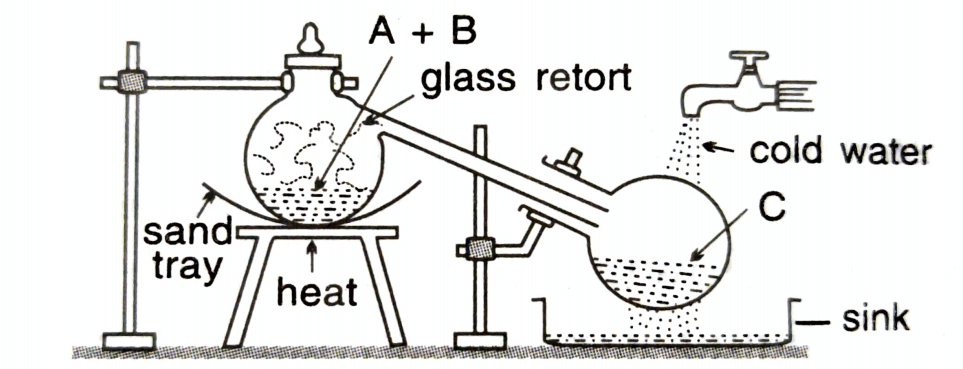1. Name A (a liquid), B (a solid), and C (a liquid). (Do not give the formulae)
2. Write an equation to show how nitric acid undergoes decomposition.
3. Write an equation for the reaction in which copper is oxidised by concentrated nitric acid.

Solution:

a. A = Conc. Sulphuric acid

B =  Sodium nitrate

C =  Nitric acid

b.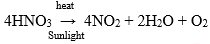c. Dilute nitric acid with copper

3Cu + 8HNO3 → 3Cu(NO3) + 4H2O + 2NO

Cu + 4HNO3 → Cu(NO3) + 2H2O + 2NO2

15.

a. A dilute acid B does not normally give hydrogen when reacted with metals but does give a gas when reacts with copper. Identify.

Write the equation with copper.

b. Complete the table:

 Name of process Inputs Equation Output Ammonia + Air Nitric acid

c. What is the property of nitric acid that allows it to react with copper?

Solution:

a. Nitric acid is dilute acid.

3Cu + 8HNO3 → 3Cu(NO3) + 4H2O + 2NO

b.

 Name of process Inputs Equation Output Ostwald process Ammonia + Air 4NH3 + 5O2 → 4NO + 6H2O + heat 2NO + O2 → 2NO2 4NO2 + 2H2O + O2 → 4HNO3 Nitric acid

c. The oxidising property of nitric acid allows it to react with copper.

16.  X, Y and Z are three crystalline solids which are soluble in water and have common anion.

To help you identify X, Y and Z, you are provided with the following experimental observations. Copy and complete the following inferences in (a) to (f).

a. A reddish-brown gas is obtained when X, Y and Z are warmed separately with concentrated sulphuric acid and copper turning added to the mixture.

Inference 1: The common anion is the _______ ion.

b. When X is heated, it melts and gives off only one gas which relights a glowing splint.

Inference 2: The cation in X is either ________ or ________.

c. The action of heat on Y produces a reddish-brown gas and yellow residue which fuses with the glass of the test tube.

Inference 3: Metal ion present in Y is the _______ ion.

d. When Z is heated, it leaves no residue. Warming Z with sodium hydroxide solution liberates a gas which turns moist red litmus paper blue.

Inference 4: Z contains _________ cation.

e. Write the equations for the following reaction:

(i)  X and conc. Sulphuric acid (below 200℃). (One equation only for either of the cations in inference 2).

(ii)   The action of heat on Y.

(iii)   Conc. nitric acid is added to copper turnings kept in a beaker.

Solution:

1. Nitrate
2. Sodium or potassium
4. Ammonia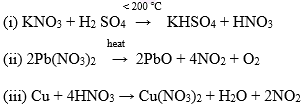17. The action of heat on blue crystalline solid X gives reddish-brown gas Y, a gas which relights a glowing splint and leaves a black residue. When gas Z, which has a rotten egg smell, is passed through a solution of X, a black ppt is formed.

1. Identify X, Y and Z.
2. Write the equation for the action of heat on X.
3. Write the equation between solution X and gas Z.

Solution:

a. X = Copper nitrate

Y = Nitrogen dioxide

Z = Hydrogen sulphate

b.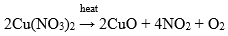c. Cu (NO3)2 + H2S → CuS + 2HNO3

To gain a deeper insight into the fundamentals of this chapter students can download concise chemistry class 10 ICSE Solutions for chapter 10 – nitric acid provided here. These solutions can help students in solving difficult questions and get familiar with all the experiments and diagrams. Students will further find all the answers to chapter questions and they refer to these solutions to complete their assignments, revisions and perform excellently in the exams.

The given solutions are as per the 2019-20 Concise Selina textbook. The Selina Solutions for the academic year 2023-24 will be updated soon.